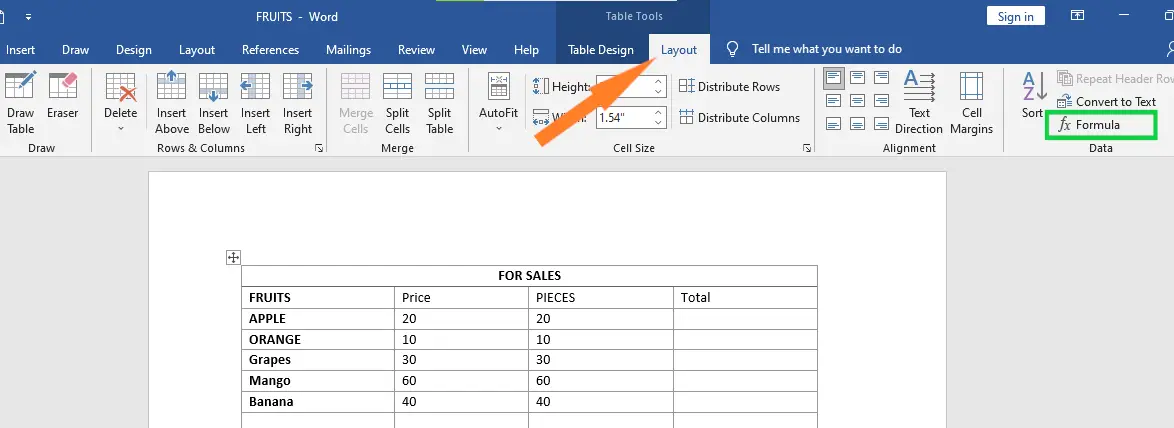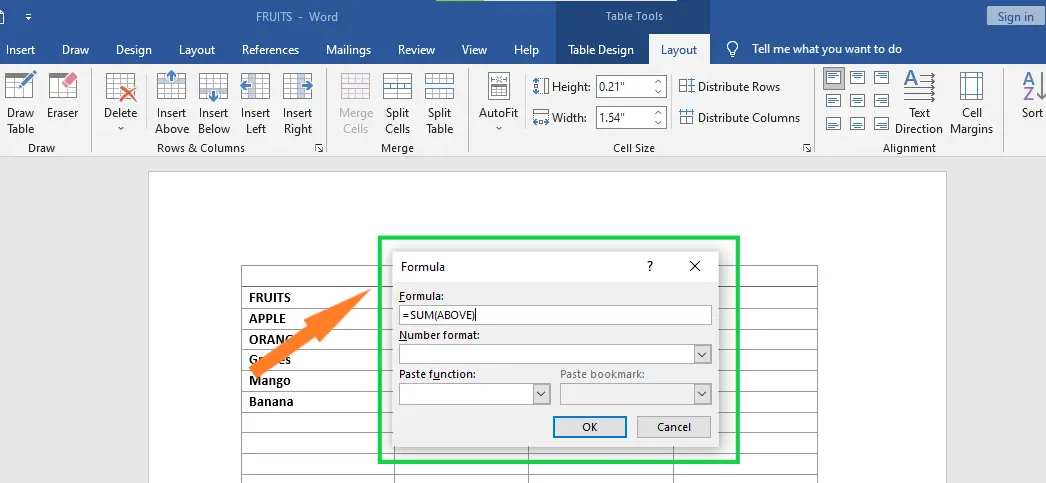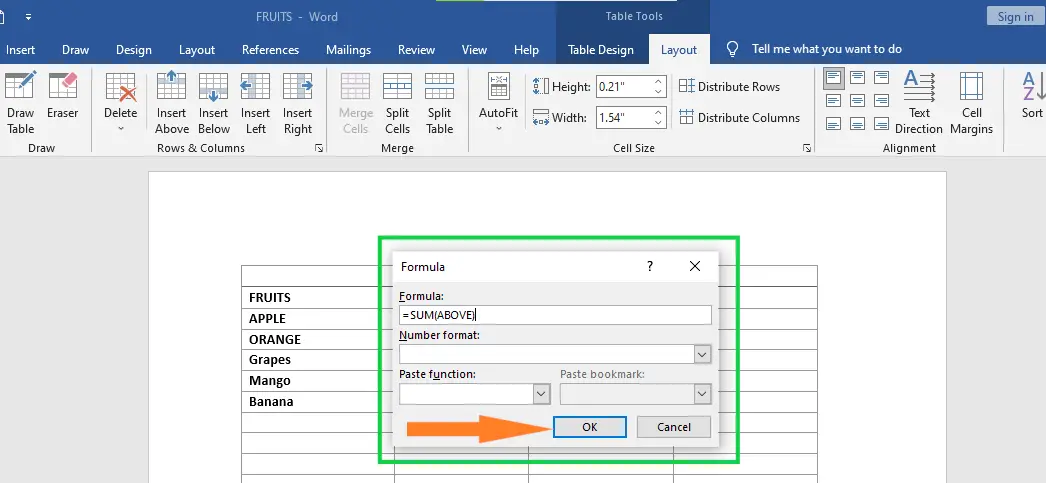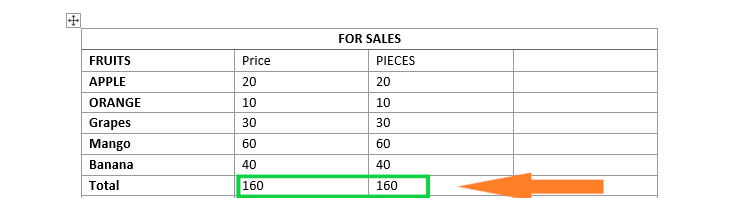# How To Add Formula To A Table In Word

In this chapter, we will discuss how to add formula to a table in Word. Microsoft Word allows you to use mathematical formula in table cells which can be used to add numbers, to find the average of numbers, or find the largest or the smallest number in table cells you specify.

There is a list of formulae that you can choose from based on the requirements. This chapter will teach you how to use formulas in word tables.

## Formula

Start your formula with an equal sign, and then type your function, such as AVERAGE, COUNT, or PRODUCT. In the parentheses, add the position of the cells you want to use for the formula. Use the positions ABOVE, BELOW, LEFT, and RIGHT.

Depending on where the cells are in relation to the formula, you can also combine positions. For example, you can use LEFT, RIGHT for cells to the left and right, or LEFT, ABOVE for cells to the left and above the cell.

## How To Add Formula To A Table In Word

Here are some easy steps for adding a formula to a table cell in a Word document.

• Step 1: First, click the tab that says Layout. Click “Formula” in the Data section on the right side of the ribbon.• Step 2: This will bring up a Formula Dialog Box with a default formula, which in our case is =SUM(LEFT).

Using the Number Format List Box, you can choose how to show the result. Using the Formula List Box, you can change the formula.• Step 3: Click OK to use the formula.• You’ll see that the above cells have been added together and the total has been put in the total cell, where we wanted it.

You can do the same thing again to get the sum of the other two rows.## List of Cell Formulae You can Use

The table shows the Formula dialog box that provides the following important functions to be used as formulas in a cell.

## Word Table Formula Cell Reference

The following are useful points to help you in constructing a word cell formula.

## Summary

In the end, we’ve learned how to add a formula to a table in Word and what those terms mean. We also know that different formulas can be used, which we can use in our document to make it look more professional and presentable.

Meanwhile, if you want to learn more about working on tables, see the previous tutorial on how to resize tables and how to merge and split tables in Microsoft Word. You can browse those guides if you are having a hard time working with your tables in a document.

We hope this tutorial helps you as you format your documents in MS Word.